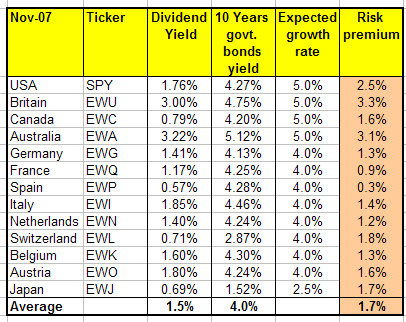November 24, 2008

One method in which examining risk premium based on implied dividend-yield level may reveal buying opportunities.

The crisis in the global stock markets brought valuation ratios to levels that were not seen in many years. Dividend yields, in many stock markets, have reached skyrocketing levels that have attracted the attention of several market observers.

What should investors conclude from such high levels of dividend yields?

One approach, which is based on the Dividend Growth Model (DGM), is to look at the risk premium that is implied by the level of the dividend yield.

According to DGM, the price of a stock is the infinite discounted sum of its future dividends. This can be presented as follows:

(1)  P = D / (r - g)

P - The price of the stock

D - The current dividend

r - The rate of return which will compensate investors for the risk of investing in equities

g - The expected long-term growth rate of dividends

Based on equation (1), we can present the rate of return that investors expect to receive in the stock market as the sum of the dividend yield and the expected growth rate of dividends:

(2)   r = D/P + g

The rate of return that investors expect to receive when they buy equities is also the sum of two components: the risk-free rate of return and the risk premium.

(3) r = Rf + RP

Rf - The risk-free rate of return

Thus, we can present the risk premium as a function of the dividend yield, the anticipated growth rate of dividends and the risk-free rate of return:

(4) RP = D/P + g - Rf

Using equation (4), it is possible to calculate the risk premium that investors attribute to equities in different stock markets. Such calculation requires an estimate of the expected long-term growth rate of dividends. A plausible assumption is that the expected long-term growth of dividends is equal to the expected nominal growth of the respective economy, e.g., the expected real growth of the economy plus the expected inflation.

Based on this assumption, I have calculated the risk premiums that were demanded by investors a year ago in stock markets of 13 developed countries. I used the five-year forecasts of inflation and GDP growth that are published by the economist intelligence unit (EIU) and the dividend yields of exchange-traded funds that follow the MSCI index of each respective country (except for the U.S., for which I used SPY, which follows the S&P 500 Index). As the risk-free rate of return, I used the yield of 10-year government bonds for each respective country. The results are presented in Table 1:

Table 1Sources: Morningstar, The Economist, EIU and author's calculations

The average dividend yield last year in the stock markets of the developed countries that were examined was 1.5%. The average implied risk premium was 1.7%, and it varied between 0.3% in Spain to 3.3% in Britain.

What's the implication of these figures on the valuation of the respective stock markets a year ago?